Connectez-vous !Cliquez ici pour vous connecter
Nouveau compte
Des millions de comptes créés.

100% gratuit !
[Avantages]Comme des milliers de personnes, recevez gratuitement chaque semaine une leçon d'anglais !

- Accueil
- Aide/Contact
- Accès rapides
- Imprimer
- Lire cet extrait
- Livre d'or
- Nouveautés
- Plan du site
- Presse
- Recommander
- Signaler un bug
- Webmasters
- Lien sur votre site

> Nos sites :
-Jeux gratuits
-Nos autres sites

Apprendre l'anglais > Cours & exercices d'anglais > Exercices d'anglais > test d'anglais n°3778 : Fractions> Plus de cours & d'exercices d'anglais sur le même thème : Nombres [Autres thèmes] > Tests similaires : - Chiffres et nombres - Nombres supérieurs à 100 - Dire/Ecrire la date en anglais - Dire la taille - Nombres - Vocabulaire : Les nombres cardinaux et ordinaux - Lire les fractions et pourcentages en anglais - Accord de hundred, thousand, million > Double-cliquez sur n'importe quel terme pour obtenir une traduction...

# Fractions

A fraction is a part of a whole, like one half (1/2), one third (1/3), two thirds (2/3), one quarter (1/4), etc. For example, half of an apple is a fraction of an apple.

A fraction can be expressed in the form a/b, where the top number, a, is called the numerator; the bottom number, b, is called the denominator. The denominator of a fraction cannot be equal to zero (this is because you cannot divide a number by zero).

The value of a fraction is not changed if both the numerator and denominator are multiplied or divided by the same non-zero number.

Equivalent Fractions :Equivalent fractions are different fractions that are equal to the same number (like 2/4 and 3/6) and can be simplified and written as the same fraction (1/2 in this example).

Expressing Fractions in Simplest Form :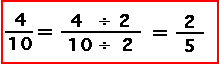A fraction is in its simplest form (this is also called being expressed in lowest terms) if the Greatest Common Factor (GCF), also called the Greatest Common Denominator (GCD), of the numerator and denominator is 1. To reduce a fraction to lowest terms (also called its simplest form), divide both the numerator and denominator by the GCD. For example, 2/3 is in lowest form, but 4/6 is not in lowest form (the GCD of 4 and 6 is 2) and 4/6 can be expressed as 2/3.

Improper Fractions :An improper fraction is one whose numerator is larger than the denominator (like 5/4). Improper fractions can be written as a mixed number, a number plus a fraction (for example, 5/4 = 1 1/4).

Fraction Written as Decimals :Every fraction can be expressed as decimal (a number with a decimal point). One way to convert a fraction to a decimal is to divide the numerator by the denominator. For example, 1/2 is equal to 1 divided by 2, which is equal to 0.5.

Fractions to Percents :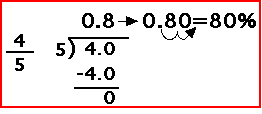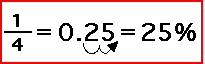A fraction can be expressed as a percentage by converting the fraction to a decimal number, then multiplying by 100 to obtain a percentage (since percent means per 100). For example, 1/2 = 0.5 = 50%.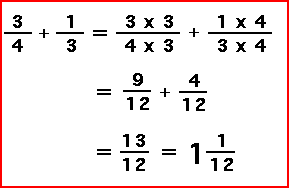To add two or more fractions, first make all the fractions have the same denominator. Once the denominators are the same, just add the numerators. Then simplify the answer (put the fraction in lowest terms).

Multiplying Fractions :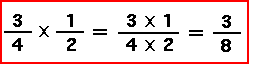To multiply two or more fractions, multiply the numerators and multiply the denominators. Then simplify the answer (put the fraction in lowest terms).

Comparing Fractions :To compare two fractions (that is, to find out which one is bigger or smaller), first write the fractions so that they have the same denominator. Then, compare the numerators; the fraction with the largest positive numerator is the larger fraction.

Exercice : Répondez aux questions en choisissant la réponse dans celles proposées.Intermédiaire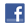Tweeter Partager
Exercice d'anglais "Fractions" créé par anonyme avec le générateur de tests - créez votre propre test !
Voir les statistiques de réussite de ce test d'anglais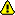Merci de vous connecter à votre compte pour sauvegarder votre résultat.

1. What is a fraction ?

2. Which number is called the numerator ?

3. Why the denominator of a fraction can't be equal to zero ?

4. When is a fraction in its simplest form ?

5. What are equivalent fractions ?

6. What is an improper fraction ?

7. How can an improper fraction be written ?

8. How convert a fraction to a decimal ?

9. How two or more fractions can be added ?

10. How can a fraction be expressed as a percentage ?

Fin de l'exercice d'anglais "Fractions"
Un exercice d'anglais gratuit pour apprendre l'anglais.
Tous les exercices | Plus de cours et d'exercices d'anglais sur le même thème : Nombres

>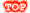INDISPENSABLES : TESTEZ VOTRE NIVEAU | GUIDE DE TRAVAIL | NOS MEILLEURES FICHES | Les fiches les plus populaires | Une leçon par email par semaine | Exercices | Aide/Contact

> INSEREZ UN PEU D'ANGLAIS DANS VOTRE VIE QUOTIDIENNE ! Rejoignez-nous gratuitement sur les réseaux :
| Cours, leçons et exercices d'anglais 100% gratuits, hors abonnement internet auprès d'un fournisseur d'accès.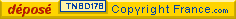| Partager sur les réseaux